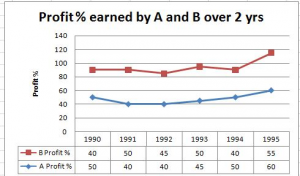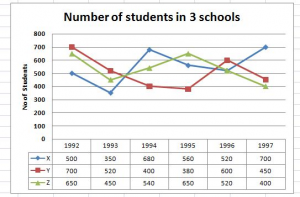## Data Interpretation For SBI PO : Set – 49

D.1-5) Study the following information to answer the given questions1) Expenditure of A in 1994 and 1995 are Rs. 12lak and Rs.14lak. What was the total income of A in 1994 and 1995 together ?

a) Rs.40.8L

b) Rs.40.4L

c) Rs.44L

d) Rs.46.4L

e) None of these

b)

1994 E = 12 L

% P = (I – E)*100 / E

50 = (I – 12)*100 / 12

I = 6+12 = 18 L

1995 E = 14L

60 = (I – 14)*100 / 14

I = 22.4L

Total = 18+22.4 = 40.4L

2) Ratio of Expenditure of Companies A and B in 1993 was 4:5. What was the ratio of their incomes in the same year ?

a) 58:75

b) 75:58

c) 78:55

d)72:55

e) None of these

a)

A : B = 40:50

45 = (I – 40)*100/40………….(A)

I = 58L

50 = (I – 50)*100/50

I = 75L ………………………………(B)

A:B = 58:75

3) Total Expenditure of company B in all the years together was Rs.125L. What was the total income of the company in all the years together ?

a) Rs.185L

b) Rs,.520L

c) Rs.250L

d) Cannot be determined

e) None of these

d)

Over all % is not known

4) If the incomes of the B in 1992 and 1993 were in the ratio 3:4, Find the ratio of Expenditures of that company in these 2 years ?

a) 48:55

b) 42:53

c) 58:45

d) 45:58

e) None of these

d)

Income => 1992:1993 = 3:4 = 30:40

45 = (30-E)*100/E

45E/100 = 30 – E

9E/20 = 30 – E

9E = 600 – 20E

29E = 600

E = 600/29……………1992

50 = (40 – E)*100 / E

50E/100 = 40 – E

E = 2(40 – E)

E = 80 – 2E

3E = 80
E = 80/3

Ratio = (600/29) / (80/3) = 600*3 / 29*80

= 1800/2320 = 180/232 = 45/58

5) If the expenditure of A and B in 1991 were equal and total income of  A and B was
Rs. 116L, what was the total expenditure of A and B in the same years ?

a) Rs.84L

b)Rs.83L

c)Rs.80L

d)Rs.40L

e) None of these

c)

For A

40 = (I – E)*100/E

40E = (I – E)100

2E = 5I – 5E

5I = 7E

I = 7/5 E

For B

50 = (116-I – E)*100/E

E = 2(116 – I – E)

E = 232 -2I – 2E

2I = 232 – 3E

I = (232 – 3E)/2

7/5E = (-232 – 3E)/2

14E = 1160  – 15E

29E = 1160

E = 1160/29 = 40

2E = 80

D.6-10) Study the following bar graph carefully to answer the given questions6) Total number of students in the School Z in the years 1992,1994 and 1997 together ?

a) 1670

b)1950

c)1590

d)1580

e) None of these

C)

Z = 650 + 540 + 400 = 1590

7) What was the approximate average no of students in School Y over all the years together ?

a) 500

b) 502

c) 506

d) 508

e) None of these

d)

Y = 700+520+400+380+600+450/ 6= 3050/6 = 508

8) What was the difference between the total no of students in all the schools together in 1993 and 1995 ?

a) 320

b) 350

c) 270

d) 250

e) None of these

c)

1993 = 1320

1995 = 1590

Diff = 270

9) Total no of students in School Y and School Z together in the year 1994 is what % less than the total no of students in School X and School Y in the year 1997 ?

a) 18.26%

b) 25.67%

c) 32.50%

d) 39.78%

e) None of these

a)

1994 = 940

1997 = 1150

(1150 – 940/1150)*100 = 18.26%

10) Number of students in the School Y is what % of the total no of students in all the three schools together in 1993 ?

a) 45%

b) 39%

c) 35%

d) 52%

e) None of these